# Mathematical Diagrams

ConceptDraw DIAGRAM diagramming and vector drawing software extended with Mathematics solution from the Science and Education area is the best for creating:

• mathematical diagrams,
• graphics,
• tape diagrams
• various mathematical illustrations

of any complexity quick and easy.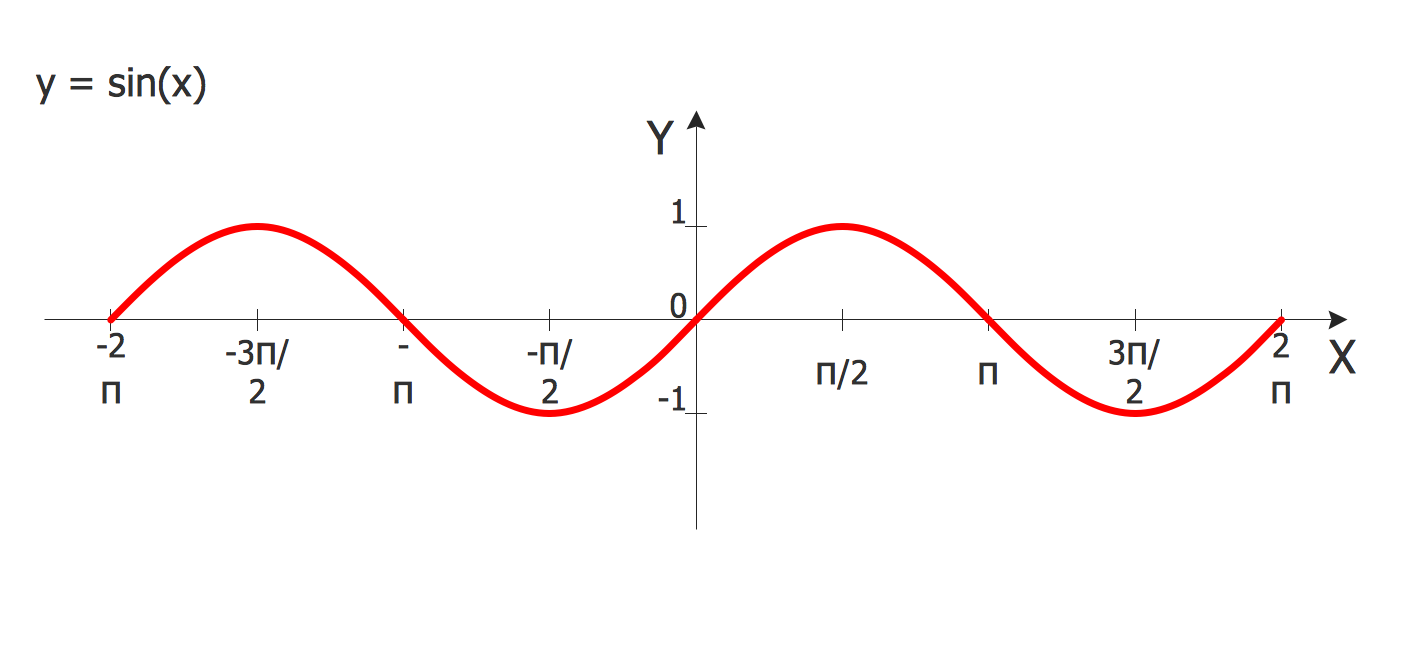Sample 1. Mathematical diagrams â€“ Sine Function

Mathematics Solution offers a set of tools for convenient and fast drawing professional looking mathematical diagrams.

Mathematics solution provides 3 libraries:

• Plane Geometry Library
• Solid Geometry Library
• Trigonometric Functions Library

with large quantity of vector shapes of plane and solid geometry figures, mathematics symbols and trigonometrical functions.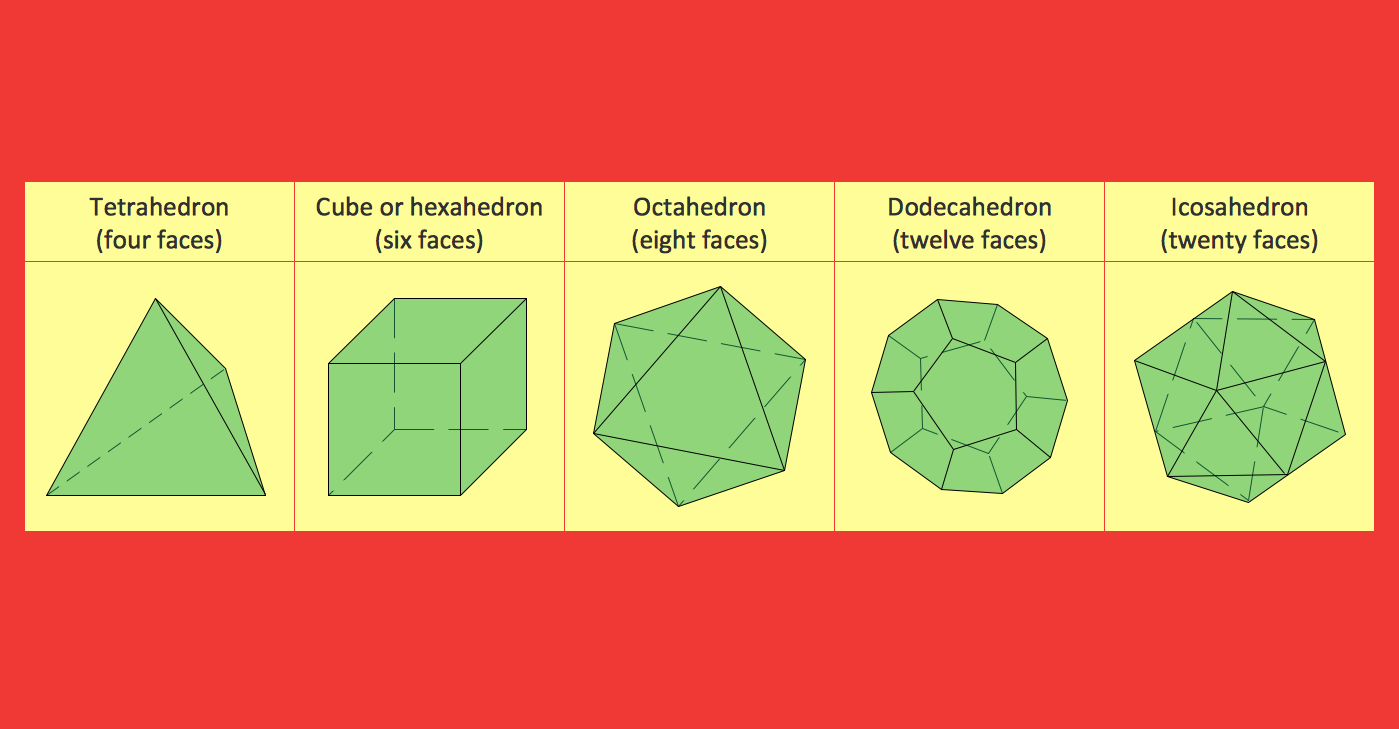Sample 2. Mathematical diagrams â€“ Platonic Solid

Mathematics solution contains also a set of different examples of mathematical diagrams. All mathematical samples and templates are available from ConceptDraw STORE and you can open them, and change for your needs.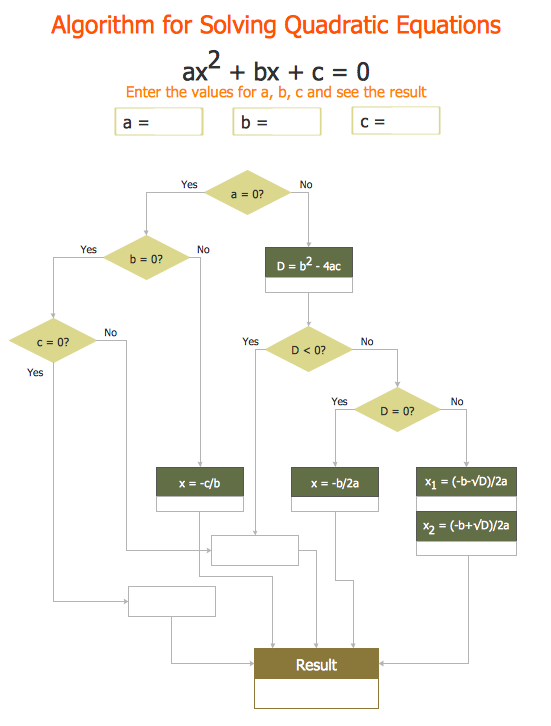Sample 3. Mathematical diagrams â€“ Solving Quadratic Equation

The samples you see on this page were created in ConceptDraw DIAGRAM using the mathematics symbols and geometry shapes from the Mathematics Solution for ConceptDraw DIAGRAM They successfully demonstrate solution's capabilities and professional results you can achieve. An experienced user spent 5 minutes creating every of these samples.

Use the Mathematics Solution for ConceptDraw DIAGRAM software to create your own professional looking mathematical diagrams and illustrations of any complexity quick, easy and effective, and then successfully use them in your scientific or education activity.

All source documents are vector graphic documents. They are available for reviewing, modifying, or converting to a variety of formats (PDF file, MS PowerPoint, MS Visio, and many other graphic formats) from the ConceptDraw STORE. The Mathematics Solution is available for all ConceptDraw DIAGRAM or later users.

Related Solution:

### Scientific Symbols Chart

ConceptDraw DIAGRAM v12 is the beautiful design software that provides many vector stencils, examples and templates for drawing different types of illustrations and diagrams.

Mathematics Solution from the Science and Education area of ConceptDraw Solution Park includes a few shape libraries of plane, solid geometric figures, trigonometrical functions and greek letters to help you create different professional looking mathematic illustrations for science and education.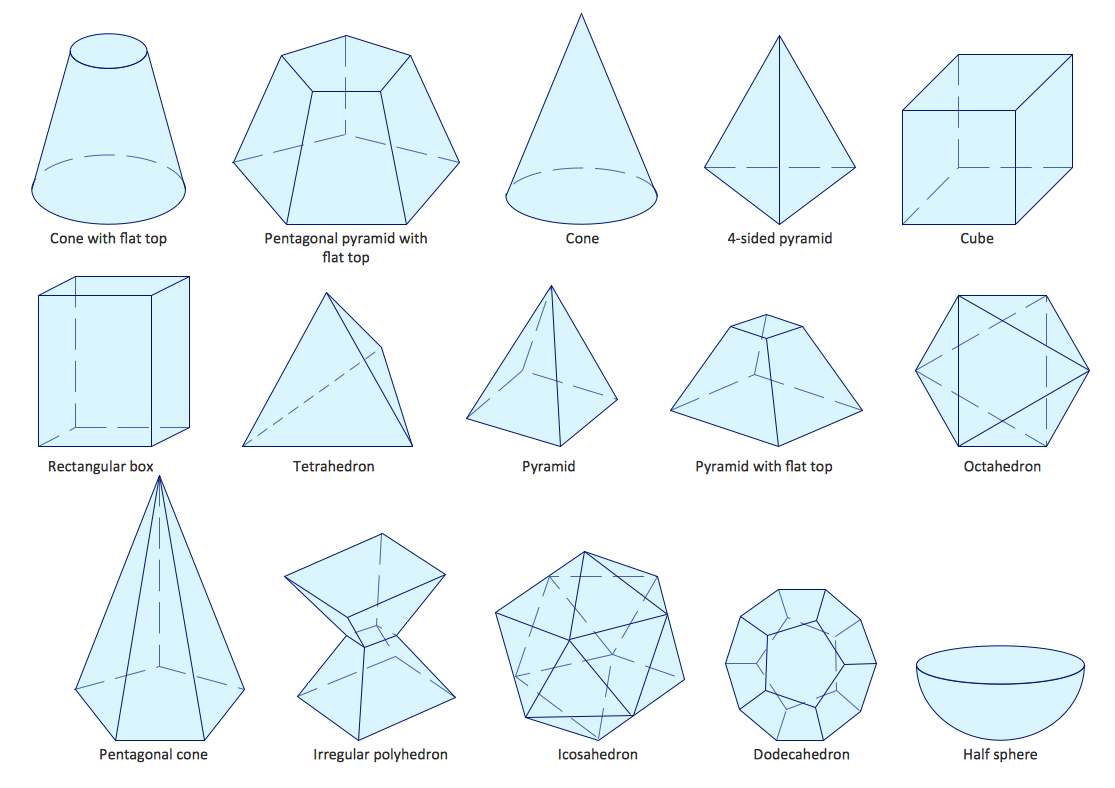Picture: Scientific Symbols Chart
Related Solution:

### Mathematics Symbols

ConceptDraw DIAGRAM v12 extended with Mathematics solution from the Science and Education area is a powerful diagramming and vector drawing software that offers all needed tools for mathematical diagrams designing. Mathematics solution provides 3 libraries with predesigned vector mathematics symbols and figures: Solid Geometry Library, Plane Geometry Library and Trigonometric Functions Library.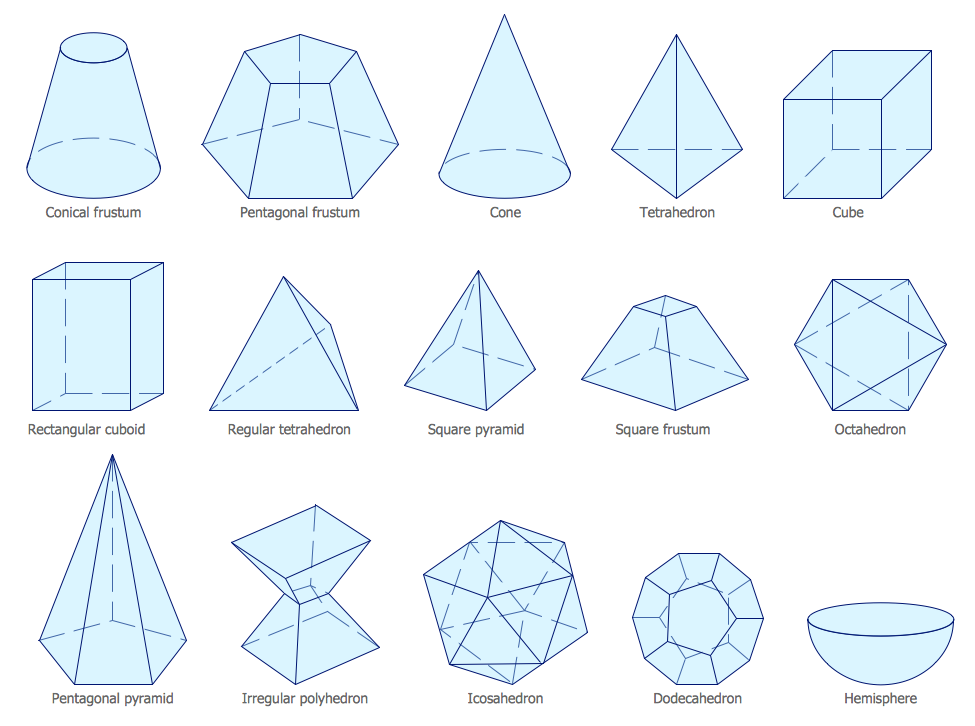Picture: Mathematics Symbols
Related Solution: